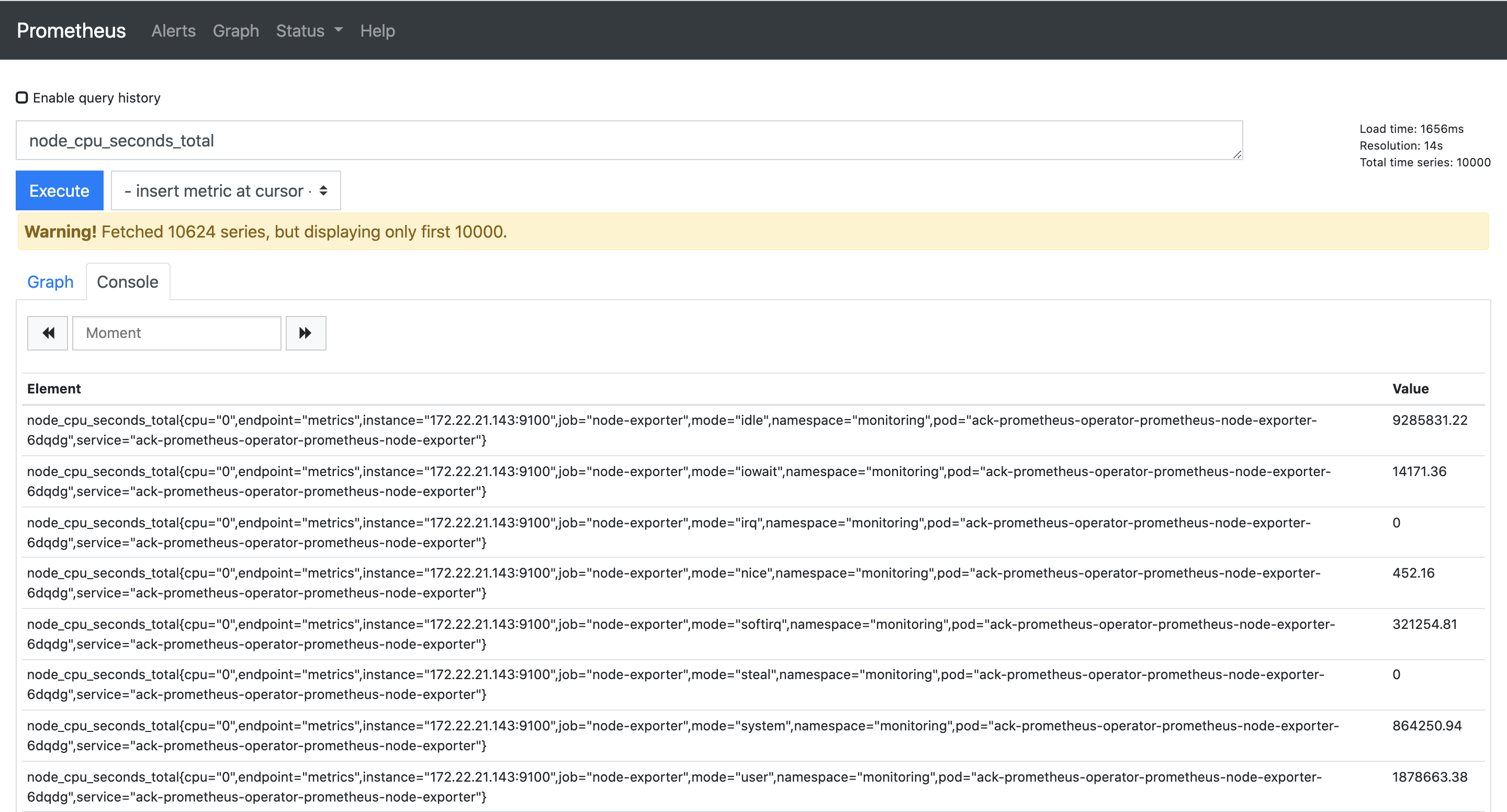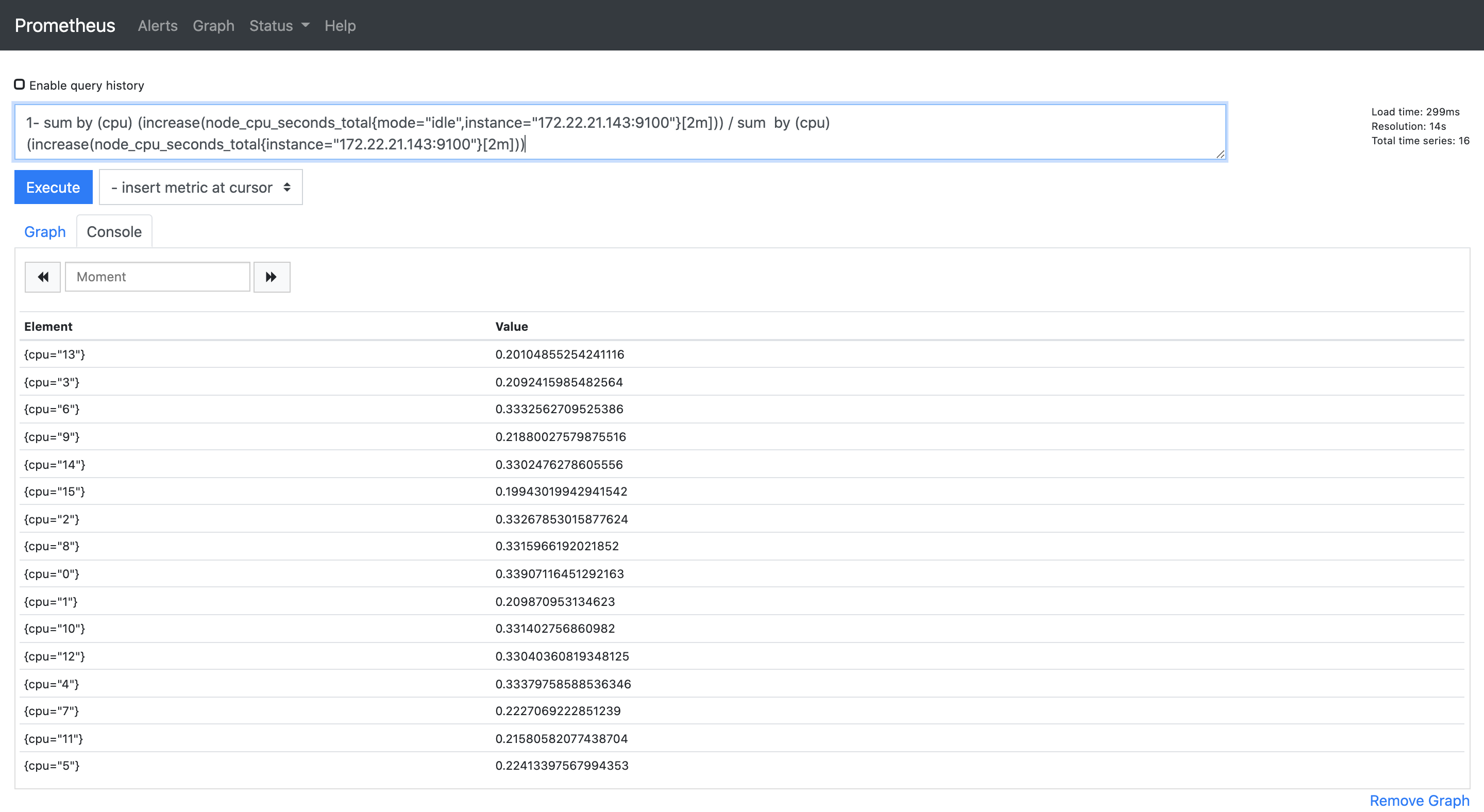# PromQL 计算 CPU 使用率

## CPU 模式

``````~# top
top - 12:13:21 up 93 days, 14:10,  1 user,  load average: 0.00, 0.02, 0.00
Tasks: 132 total,   1 running, 131 sleeping,   0 stopped,   0 zombie
%Cpu(s):  1.0 us,  0.3 sy,  0.0 ni, 98.7 id,  0.0 wa,  0.0 hi,  0.0 si,  0.0 st
``````

• us: user 模式
• sy: system 模式
• ni: nice 模式
• id: idle 模式
• wa: iowait 模式
• hi: hardware interrupt 模式
• si: software interrupt 模式
• st: steal 模式

## CPU 时间## 计算 CPU 使用率

``````CPU 使用率 = (1 - 所有空闲状态 CPU 时间总和 / 所有状态 CPU 时间总和) * 100%
``````

``````increase(node_cpu_seconds_total{cpu="0",instance="172.22.21.143:9100",mode="idle"}[2m])
````````````1 - increase(node_cpu_seconds_total{cpu="0",instance="172.22.21.143:9100",mode="idle"}[2m]) / 120
````````````1 - sum(increase(node_cpu_seconds_total{cpu="0",instance="172.22.21.143:9100",mode="idle"}[2m])) / sum(increase(node_cpu_seconds_total{cpu="0",instance="172.22.21.143:9100"}[2m]))
``````

``````sum(increase(node_cpu_seconds_total{cpu="0",instance="172.22.21.143:9100"}[2m]))
``````

``````1 - rate(node_cpu_seconds_total{cpu="0",instance="172.22.21.143:9100",mode="idle"}[2m])
````````````1 - sum by (cpu) (increase(node_cpu_seconds_total{mode="idle",instance="172.22.21.143:9100"}[2m])) / sum by (cpu) (increase(node_cpu_seconds_total{instance="172.22.21.143:9100"}[2m]))
````````````1 - sum (increase(node_cpu_seconds_total{mode="idle",instance="172.22.21.143:9100"}[2m])) / sum (increase(node_cpu_seconds_total{instance="172.22.21.143:9100"}[2m]))
````````````1 - sum by (instance)(increase(node_cpu_seconds_total{mode="idle"}[2m])) / sum by (instance)(increase(node_cpu_seconds_total[2m]))
````````````1 - (avg by(instance) (rate(node_cpu_seconds_total{mode="idle"}[2m])))
``````